Electron. J. Diff. Eqns., Vol. 2000(2000), No. 10, pp. 1-19.

### A second eigenvalue bound for the Dirichlet Schrodinger equation wtih a radially symmetric potential Craig Haile

Abstract:
We study the time-independent Schrodinger equation with radially symmetric potential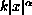,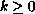,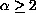on a bounded domain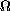in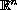,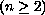with Dirichlet boundary conditions. In particular, we compare the eigenvalue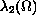of the operator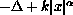onwith the eigenvalue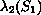of the same operator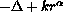on a ball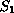, wherehas radius such that the first eigenvalues are the same,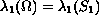. The main result is to show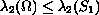. We also give an extension of the main result to the case of a more general elliptic eigenvalue problem on a bounded domain with Dirichlet boundary conditions.

Submitted August 24, 1999. Published Janaury 28, 2000.
Math Subject Classifications: 35J10, 35J15, 35J25, 35P15.
Key Words: Schrodinger eigenvalue equation, Dirichlet boundary conditions, eigenvalue bounds, radially symmetric potential.

Show me the PDF file (194K), TEX file, and other files for this article.Craig Haile Department of Mathematics and Physics College of the Ozarks Point Lookout, MO 65726-0017, USA e-mail: haile@cofo.edu